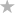#### File Exchange > Data Analysis >    Effect Size for ANOVAAuthor:
OriginLab Technical Support
5/31/2022
Last Update:
11/3/2022
164
Total Ratings:
1
File Size:
145 KB
Average Rating:File Name:
Effect_Siz...VA.opx
File Version:
1.00
Minimum Versions:
Summary:

Calculate effect size from ANOVA results.

Description:

PURPOSE
This app can be used to calculate effect size from ANOVA analysis report.

INSTALLATION
Download the file Effect_Size_for_ANOVA.opx, and then drag-and-drop onto the Origin workspace. An icon will appear in the Apps Gallery window.
NOTE: This tool requires OriginPro.

OPERATION

1. Make a report sheet with ANOVA table active. Report sheet can be created by Two-Way ANOVA, Three-Way ANOVA or General Linear Regression app.Click on the Effect Size for ANOVA icon in the Apps Gallery window. A dialog will open.
2. Choose results to calculate in Quantities group. Four options are available: Eta-squared, Partial Eta-squared, Omega-squared, and Partial Omega-squared. Click OK button. A report sheet with Effect Size table is created.

SAMPLE OPJU FILE
This app provides a sample OPJU file. Right click on the Effect Size for ANOVA icon in the Apps Gallery window, and choose Show Samples Folder from the short-cut menu. A folder will open. Drag-and-drop the project file EffectSizeEx.opju from the folder onto Origin. The Notes window in the project shows detailed steps.
Note: If you wish to save the OPJU after changing, it is recommended that you save to a different folder location (e.g. User Files Folder).

ALGORITHM

• Eta-squared
$$\eta^2 = \frac{SS_{effect}}{SS_{total}}$$
• Partial Eta-squared
$$\eta_p^2 = \frac{SS_{effect}}{ SS_{effect} + SS_{error} }$$
• Omega-squared
$$\omega^2 = \frac{ SS_{effect} - df_{effect} MS_{error} }{ MS_{error} + SS_{total} }$$
• Partial Omega-squared
$$\omega_p^2 = \frac{ SS_{effect} - df_{effect} MS_{error} }{ SS_{effect} + (df_{total}+1-df_{effect}) MS_{error} }$$
where SS, df and MS are sum of squares, degrees of freedom and mean square respectively in the ANOVA table.

REFERENCE

1. Kelley, T. (1935) "An unbiased correlation ratio measure." Proceedings of the National Academy of Sciences. 21(9). 554-559.
2. effectsize package in R